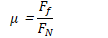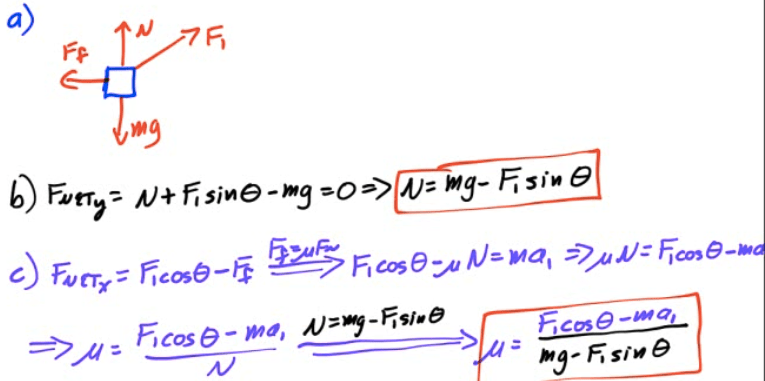# Coefficient of Friction• Ratio of the frictional force and the normal force provides the coefficient of friction

•# Kinetic or Static# Calculating the Force of Friction

• The force of friction depends only upon the nature of the surface in contact (μ) and magnitude of the normal force FN

• Combine with Newton's Second Las and FBDs to solve more involved problems

# 2007 Free Response Question 1